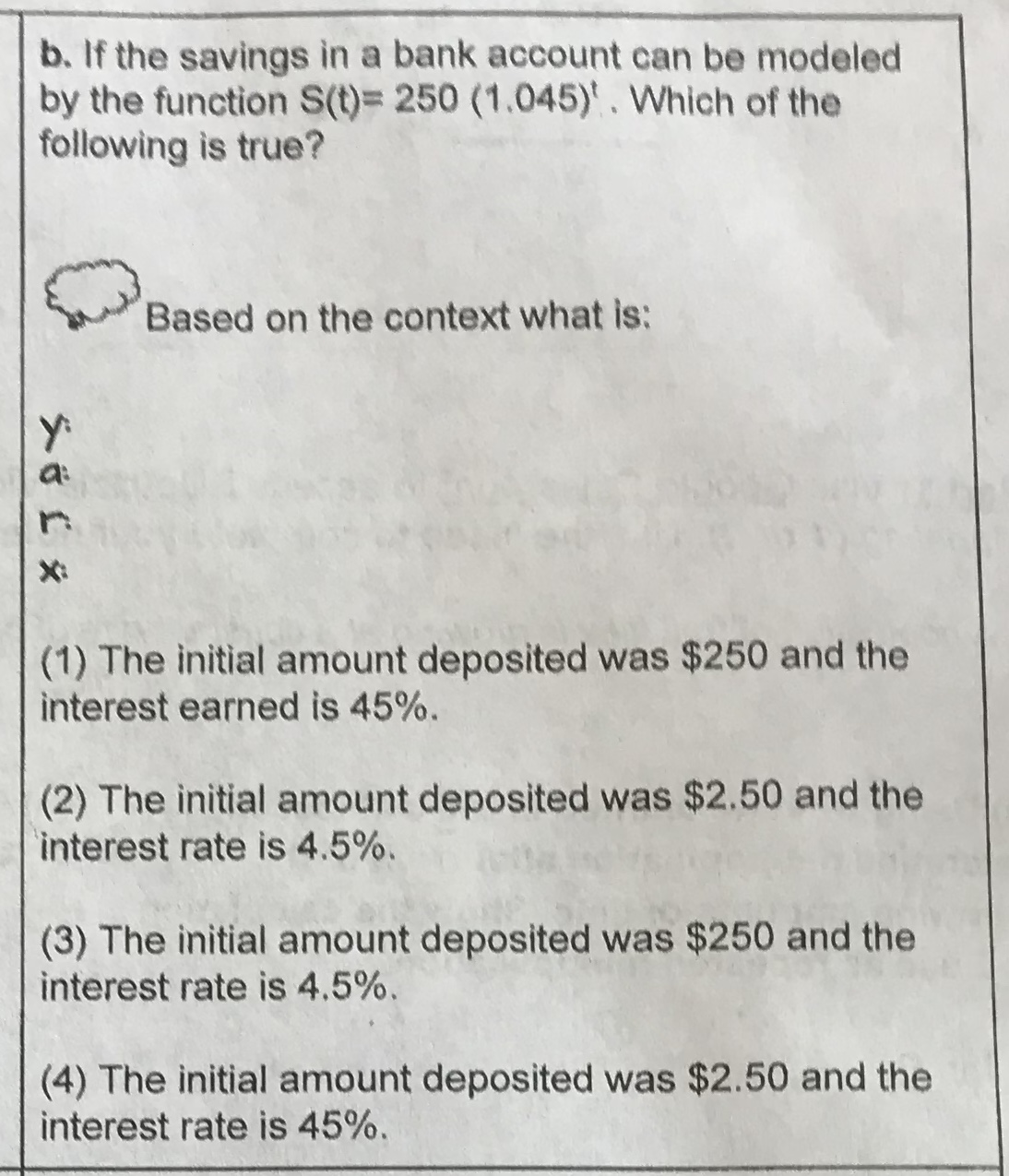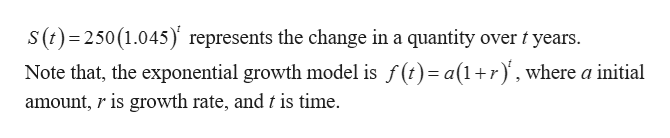# b. If the savings in a bank account can be modeledby the function S(t) 250 (1.045)'. Which of thefollowing is true?Based on the context what is:X(1) The initial amount deposited was \$250 and theinterest earned is 45%(2) The initial amount deposited was \$2.50 and theinterest rate is 4.5%.(3) The initial amount deposited was \$250 and theinterest rate is 4.5%.(4) The initial amount deposited was \$2.50 and theinterest rate is 45%.

Question
20 viewshelp_outlineImage Transcriptioncloseb. If the savings in a bank account can be modeled by the function S(t) 250 (1.045)'. Which of the following is true? Based on the context what is: X (1) The initial amount deposited was \$250 and the interest earned is 45% (2) The initial amount deposited was \$2.50 and the interest rate is 4.5%. (3) The initial amount deposited was \$250 and the interest rate is 4.5%. (4) The initial amount deposited was \$2.50 and the interest rate is 45%. fullscreen
check_circle

Step 1

The given funct...help_outlineImage Transcriptioncloses (t) 250 (1.045)' represents the change in a quantity over t years. Note that, the exponential growth model is f(t)= a(1r, where a initial amount, r is growth rate, andt is time. fullscreen

### Want to see the full answer?

See Solution

#### Want to see this answer and more?

Solutions are written by subject experts who are available 24/7. Questions are typically answered within 1 hour.*

See Solution
*Response times may vary by subject and question.
Tagged in

### Other